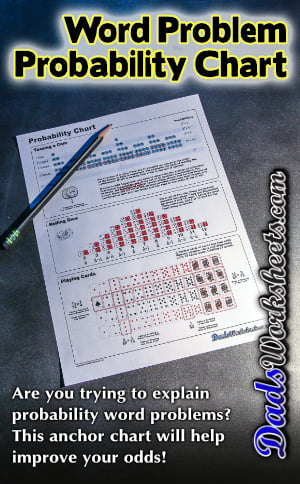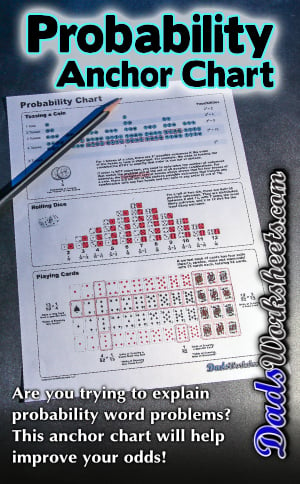PLEASE GO BACK AND USE THE BIG BLUE 'PRINT' BUTTON ON THE PAGE TO PRINT THE WORKSHEET CORRECTLY!Sorry for the trouble! The browser won't print the embedded worksheet PDF directly using the normal 'Print' command in the file menu, so you need to click the big 'Print' button to send just the worksheet and not the surrounding page to the printer.

# Probability Chart

Probability anchor chart for word problem reference! This illustrated chart describes scenarios with coins, dice and playing cards. It includes odds for most likely and least likely outcomes.

## Probability Chart for Word Problems

Probability problems can be tricky for kids, and many of the devices we use to communicate probability may initially be unfamiliar to kids. We take for granted that kids understand the idea of tossing a coin or picking a specific card from a deck of playing cards, however many kids will be unfamiliar with some of these common activities.

### Probability for Coin Tosses

A common type of probability word problem involves calculating the odds of results from multiple coin tosses. The probability chart on this page breaks down how many possible outcomes there are from a given number of coin tosses and gives the odds of a specific sequence of heads or tails outcomes occurring. It also discusses probabilities where a series of coin tosses might generate an outcome regardless of the order of the results.

### Probability for Rolling Dice

Many word problems involve calculating the probability of rolling a pair of six sided game dice. This probability chart gives the probability of all of the sums you can roll with a pair of dice. The chart illustrates the dice as a single white die and a single red die to emphasize the way the probability is calculated. Some students may be confused by the idea that rolling a three and a four is distinct from rolling a four and three (and therefore increases the odds of rolling a sum of seven.) To help explain this, the two colored die can help kids understand that order of the rolls and the individual die play a role (pardon the pun) in how the probabilities stack up.

You can further elaborate on this by explaining how getting a result on one die, and then rolling the second die, creates a distinct set of outcomes.

### Probability for Playing Cards

Playing cards are a common feature of many probability word problems, but you may be surprised at how many kids haven't been exposed to a traditional deck of 52 cards at home. This probability chart breaks down the composition of a deck of cards can gives probability for individual cards, face cards, suits and more.